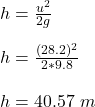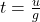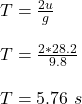## A ball is thrown upward with a speed of 28.2 m/s.A. What is its maximum height?B. How long is the ball in the air?C. When does the ball have

Question

A ball is thrown upward with a speed of 28.2 m/s.A. What is its maximum height?B. How long is the ball in the air?C. When does the ball have a speed of 12m/s?

in progress 0
7 months 2021-07-30T07:41:41+00:00 1 Answers 5 views 0

(A) The maximum height of the ball is 40.57 m

(B) Time spent by the ball on air is 5.76 s

(C) at 33.23 m the speed will be 12 m/s

Explanation:

Given;

initial velocity of the ball, u = 28.2 m/s

(A) The maximum height

At maximum height, the final velocity, v = 0

v² = u² -2gh

u² = 2gh(B) Time spent by the ball on air

Time of flight = Time to reach maximum height + time to hit ground.

Time to reach maximum height = time to hit ground.

Time to reach maximum height  is given by;

v = u – gt

u = gtTime of flight, T = 2t(C) the position of the ball at 12 m/s

As the ball moves upwards, the speed drops, then the height of the ball when the speed drops to 12m/s will be calculated by applying the equation below.

v² = u² – 2gh

12² = 28.2² – 2(9.8)h

12² – 28.2² = – 2(9.8)h

-651.24 = -19.6h

h = 651.24 / 19.6

h = 33.23 m

Thus, at 33.23 m the speed will be 12 m/s